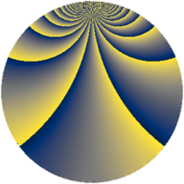# Properties

 Label 1045.1.wLevel $1045$ Weight $1$ Character orbit 1045.w Rep. character $\chi_{1045}(284,\cdot)$ Character field $\Q(\zeta_{10})$ Dimension $40$ Newform subspaces $6$ Sturm bound $120$ Trace bound $7$

# Related objects

## Defining parameters

 Level: $$N$$ $$=$$ $$1045 = 5 \cdot 11 \cdot 19$$ Weight: $$k$$ $$=$$ $$1$$ Character orbit: $$[\chi]$$ $$=$$ 1045.w (of order $$10$$ and degree $$4$$) Character conductor: $$\operatorname{cond}(\chi)$$ $$=$$ $$1045$$ Character field: $$\Q(\zeta_{10})$$ Newform subspaces: $$6$$ Sturm bound: $$120$$ Trace bound: $$7$$

## Dimensions

The following table gives the dimensions of various subspaces of $$M_{1}(1045, [\chi])$$.

Total New Old
Modular forms 56 56 0
Cusp forms 40 40 0
Eisenstein series 16 16 0

The following table gives the dimensions of subspaces with specified projective image type.

$$D_n$$ $$A_4$$ $$S_4$$ $$A_5$$
Dimension 40 0 0 0

## Trace form

 $$40 q - 6 q^{4} - 2 q^{5} - 6 q^{9} + O(q^{10})$$ $$40 q - 6 q^{4} - 2 q^{5} - 6 q^{9} + 2 q^{11} - 10 q^{16} - 2 q^{19} + 2 q^{20} - 16 q^{24} - 10 q^{25} - 16 q^{26} - 16 q^{30} - 5 q^{35} + 14 q^{36} + 2 q^{45} + 64 q^{54} - 3 q^{55} - 6 q^{61} - 6 q^{64} - 8 q^{66} - 8 q^{76} + 27 q^{80} - 10 q^{81} - 5 q^{85} - 5 q^{95} + 8 q^{96} - 10 q^{99} + O(q^{100})$$

## Decomposition of $$S_{1}^{\mathrm{new}}(1045, [\chi])$$ into newform subspaces

Label Dim $A$ Field Image CM RM Traces $q$-expansion
$a_{2}$ $a_{3}$ $a_{5}$ $a_{7}$
1045.1.w.a $4$ $0.522$ $$\Q(\zeta_{10})$$ $D_{5}$ $$\Q(\sqrt{-95})$$ None $$-2$$ $$-2$$ $$-1$$ $$0$$ $$q+(\zeta_{10}^{2}+\zeta_{10}^{4})q^{2}+(-\zeta_{10}+\zeta_{10}^{2}+\cdots)q^{3}+\cdots$$
1045.1.w.b $4$ $0.522$ $$\Q(\zeta_{10})$$ $D_{10}$ $$\Q(\sqrt{-19})$$ None $$0$$ $$0$$ $$-1$$ $$-5$$ $$q+\zeta_{10}q^{4}-\zeta_{10}q^{5}+(-1+\zeta_{10}^{2}+\cdots)q^{7}+\cdots$$
1045.1.w.c $4$ $0.522$ $$\Q(\zeta_{10})$$ $D_{10}$ $$\Q(\sqrt{-19})$$ None $$0$$ $$0$$ $$-1$$ $$5$$ $$q+\zeta_{10}q^{4}-\zeta_{10}^{3}q^{5}+(1-\zeta_{10}^{2})q^{7}+\cdots$$
1045.1.w.d $4$ $0.522$ $$\Q(\zeta_{10})$$ $D_{5}$ $$\Q(\sqrt{-95})$$ None $$2$$ $$2$$ $$-1$$ $$0$$ $$q+(-\zeta_{10}^{2}-\zeta_{10}^{4})q^{2}+(\zeta_{10}-\zeta_{10}^{2}+\cdots)q^{3}+\cdots$$
1045.1.w.e $8$ $0.522$ $$\Q(\zeta_{20})$$ $D_{10}$ $$\Q(\sqrt{-95})$$ None $$0$$ $$0$$ $$-2$$ $$0$$ $$q+(-\zeta_{20}^{3}-\zeta_{20}^{9})q^{2}+(\zeta_{20}^{7}+\zeta_{20}^{9}+\cdots)q^{3}+\cdots$$
1045.1.w.f $16$ $0.522$ $$\Q(\zeta_{40})$$ $D_{20}$ $$\Q(\sqrt{-95})$$ None $$0$$ $$0$$ $$4$$ $$0$$ $$q+(\zeta_{40}+\zeta_{40}^{7})q^{2}+(\zeta_{40}^{11}+\zeta_{40}^{13}+\cdots)q^{3}+\cdots$$